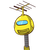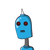# the area between a concentric circle is 1540cm². If the radius of the outer circle is 21 cm, calculate the radius of the inner cir

the area between a concentric circle is 1540cm². If the radius of the outer circle is 21 cm, calculate the radius of the inner circle​

### 2 thoughts on “the area between a concentric circle is 1540cm². If the radius of the outer circle is 21 cm, calculate the radius of the inner cir”

1.Correct Question:

The area enclosed between the concentric circles is 770 cm². If the radius of the outer circle is 21 cm, find the radius of the inner circle.

The radius of the inner circle is 14 cm.

Step-by-step explanation:

Given that:

The area between a concentric circle is 770 cm².

The radius of the outer circle is 21 cm.

To Find:

The radius of the inner circle.

Let us assume:

The radius of the inner circle be x.

Formula:

Area between a concentric circle = π(R² – r²)

Where,

R = The radius of the outer circle

r = The radius of the inner circle

Finding the radius of the inner circle:

According to the question.

⟶ π(21² – x²) = 770

⟶ π(441 – x²) = 770

⟶ 441 – x² = 770/π

⟶ 441 – x² = (770 × 7)/22

⟶ 441 – x² = 245

⟶ x² = 441 – 245

⟶ x² = 196

⟶ x = √196

⟶ x = 14

∴ The radius of the inner circle = 14 cm

2.[tex]{\textsf{\textbf{\underline{\underline{∴ The radius of the inner circle = 14 cm\::}}}}} \\ [/tex]

correct Question:

• The area enclosed between the concentric circles is 770 cm². If the radius of the outer circle is 21 cm, find the radius of the inner circle.

Step-by-step explanation:

Given that:

• The area between a concentric circle is 770 cm².
• The radius of the outer circle is 21 cm.

To Find:

• The radius of the inner circle.

Let us assume:

• The radius of the inner circle be x.

Formulas used:

• Area between a concentric circle = π(R² – r²)

Where,

• R = The radius of the outer circle
• r = The radius of the inner circle

Finding the radius of the inner circle:

According to the question.

⟶ π(21² – x²) = 770

⟶ π(441 – x²) = 770

⟶ 441 – x² = 770/π

⟶ 441 – x² = (770 × 7)/22

⟶ 441 – x² = 245

⟶ x² = 441 – 245

⟶ x² = 196

⟶ x = √196

⟶ x = 14

[tex]\small{\textsf{\textbf{\underline{\underline{∴ The radius of the inner circle = 14 cm\::}}}}} \\ [/tex]